# modelDiscrimination

Compute AUROC and ROC data

## Syntax

``DiscMeasure = modelDiscrimination(eadModel,data)``
``[DiscMeasure,DiscData] = modelDiscrimination(___,Name=Value)``

## Description

example

````DiscMeasure = modelDiscrimination(eadModel,data)` computes the area under the receiver operating characteristic curve (AUROC). `modelDiscrimination` supports segmentation and comparison against a reference model and alternative methods to discretize the EAD response into a binary variable.```

example

````[DiscMeasure,DiscData] = modelDiscrimination(___,Name=Value)` specifies options using one or more name-value arguments in addition to the input arguments in the previous syntax.```

## Examples

collapse all

This example shows how to use `fitEADModel` to create a `Tobit` model and then use `modelDiscrimination` to compute AUROC and ROC.

```load EADData.mat head(EADData)```
``` UtilizationRate Age Marriage Limit Drawn EAD _______________ ___ ___________ __________ __________ __________ 0.24359 25 not married 44776 10907 44740 0.96946 44 not married 2.1405e+05 2.0751e+05 40678 0 40 married 1.6581e+05 0 1.6567e+05 0.53242 38 not married 1.7375e+05 92506 1593.5 0.2583 30 not married 26258 6782.5 54.175 0.17039 54 married 1.7357e+05 29575 576.69 0.18586 27 not married 19590 3641 998.49 0.85372 42 not married 2.0712e+05 1.7682e+05 1.6454e+05 ```
```rng('default'); NumObs = height(EADData); c = cvpartition(NumObs,'HoldOut',0.4); TrainingInd = training(c); TestInd = test(c);```

Select Model Type

Select a model type for `Tobit` or `Regression`.

`ModelType =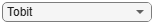"Tobit";`

Select Conversion Measure

Select a conversion measure for the EAD response values.

`ConversionMeasure =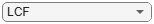"LCF";`

Create `Tobit` EAD Model

Use `fitEADModel` to create a `Tobit` model using the `TrainingInd` data.

```eadModel = fitEADModel(EADData(TrainingInd,:),ModelType,PredictorVars={'UtilizationRate','Age','Marriage'}, ... ConversionMeasure=ConversionMeasure,DrawnVar="Drawn",LimitVar="Limit",ResponseVar="EAD"); disp(eadModel);```
``` Tobit with properties: CensoringSide: "both" LeftLimit: 0 RightLimit: 1 ModelID: "Tobit" Description: "" UnderlyingModel: [1x1 risk.internal.credit.TobitModel] PredictorVars: ["UtilizationRate" "Age" "Marriage"] ResponseVar: "EAD" LimitVar: "Limit" DrawnVar: "Drawn" ConversionMeasure: "lcf" ```

Display the underlying model. The underlying model's response variable is the transformation of the EAD response data. Use the `'LiimitVar'` and `'DrwanVar'` name-value arguments to modify the transformation.

`disp(eadModel.UnderlyingModel);`
```Tobit regression model: EAD_lcf = max(0,min(Y*,1)) Y* ~ 1 + UtilizationRate + Age + Marriage Estimated coefficients: Estimate SE tStat pValue __________ __________ ________ __________ (Intercept) 0.22467 0.031679 7.092 1.6924e-12 UtilizationRate 0.4714 0.020683 22.791 0 Age -0.0014209 0.00077072 -1.8436 0.065356 Marriage_not married -0.010542 0.015811 -0.66676 0.50498 (Sigma) 0.3618 0.0049791 72.665 0 Number of observations: 2627 Number of left-censored observations: 0 Number of uncensored observations: 2626 Number of right-censored observations: 1 Log-likelihood: -1057.9 ```

EAD prediction operates on the underlying compact statistical model and then transforms the predicted values back to the EAD scale. You can specify the `predict` function with different options for the `'ModelLevel'` name-value argument.

```predictedEAD = predict(eadModel,EADData(TestInd,:),ModelLevel="ead"); predictedConversion = predict(eadModel,EADData(TestInd,:),ModelLevel="ConversionMeasure");```

For model validation, use `modelDiscrimination`, `modelDiscriminationPlot`, `modelAccuracy`, and `modelAccuracyPlot`.

Use `modelDiscrimination` and then `modelDiscriminationPlot` to plot the ROC curve.

```ModelLevel =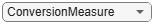"ConversionMeasure"; [DiscMeasure1,DiscData1] = modelDiscrimination(eadModel,EADData(TestInd,:),ShowDetails=true,ModelLevel=ModelLevel)```
```DiscMeasure1=1×3 table AUROC Segment SegmentCount _______ __________ ____________ Tobit 0.70893 "all_data" 1751 ```
```DiscData1=1534×3 table X Y T __________ _________ _______ 0 0 0.63602 0 0.0027778 0.63602 0 0.0041667 0.63489 0.00096993 0.0055556 0.63377 0.00096993 0.0069444 0.63265 0.0019399 0.0083333 0.63152 0.0029098 0.0097222 0.6304 0.0029098 0.015278 0.62927 0.0029098 0.016667 0.62922 0.0029098 0.018056 0.6288 0.0029098 0.019444 0.62864 0.0038797 0.022222 0.62814 0.0038797 0.025 0.62767 0.0048497 0.026389 0.62701 0.0048497 0.033333 0.62654 0.0058196 0.033333 0.62618 ⋮ ```
`modelDiscriminationPlot(eadModel,EADData(TestInd,:),ModelLevel=ModelLevel,SegmentBy="Marriage");`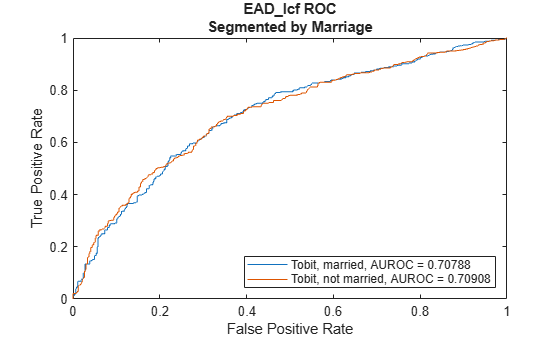This example shows how to use `fitEADModel` to create a `Beta` model and then use `modelDiscrimination` to compute AUROC and ROC.

```load EADData.mat head(EADData)```
``` UtilizationRate Age Marriage Limit Drawn EAD _______________ ___ ___________ __________ __________ __________ 0.24359 25 not married 44776 10907 44740 0.96946 44 not married 2.1405e+05 2.0751e+05 40678 0 40 married 1.6581e+05 0 1.6567e+05 0.53242 38 not married 1.7375e+05 92506 1593.5 0.2583 30 not married 26258 6782.5 54.175 0.17039 54 married 1.7357e+05 29575 576.69 0.18586 27 not married 19590 3641 998.49 0.85372 42 not married 2.0712e+05 1.7682e+05 1.6454e+05 ```
```rng('default'); NumObs = height(EADData); c = cvpartition(NumObs,'HoldOut',0.4); TrainingInd = training(c); TestInd = test(c);```

Select Model Type

Select a model type for a `Beta` model.

`ModelType =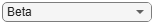"Beta";`

Select Conversion Measure

Select a conversion measure for the EAD response values.

`ConversionMeasure ="LCF";`

Create `Beta` EAD Model

Use `fitEADModel` to create a `Beta` model using the `TrainingInd` data.

```eadModel = fitEADModel(EADData(TrainingInd,:),ModelType,PredictorVars={'UtilizationRate','Age','Marriage'}, ... ConversionMeasure=ConversionMeasure,DrawnVar="Drawn",LimitVar="Limit",ResponseVar="EAD"); disp(eadModel);```
``` Beta with properties: BoundaryTolerance: 1.0000e-07 ModelID: "Beta" Description: "" UnderlyingModel: [1x1 risk.internal.credit.BetaModel] PredictorVars: ["UtilizationRate" "Age" "Marriage"] ResponseVar: "EAD" LimitVar: "Limit" DrawnVar: "Drawn" ConversionMeasure: "lcf" ```

Display the underlying model. The underlying model's response variable is the transformation of the EAD response data. Use the `'LiimitVar'` and `'DrwanVar'` name-value arguments to modify the transformation.

`disp(eadModel.UnderlyingModel);`
```Beta regression model: logit(EAD_lcf) ~ 1_mu + UtilizationRate_mu + Age_mu + Marriage_mu log(EAD_lcf) ~ 1_phi + UtilizationRate_phi + Age_phi + Marriage_phi Estimated coefficients: Estimate SE tStat pValue __________ _________ ________ __________ (Intercept)_mu -0.65566 0.11484 -5.7093 1.2616e-08 UtilizationRate_mu 1.7014 0.078094 21.787 0 Age_mu -0.0055901 0.0027603 -2.0252 0.042949 Marriage_not married_mu -0.012577 0.052098 -0.24141 0.80926 (Intercept)_phi -0.50131 0.094625 -5.2979 1.2686e-07 UtilizationRate_phi 0.39731 0.066707 5.956 2.9303e-09 Age_phi -0.001167 0.0023161 -0.50387 0.6144 Marriage_not married_phi -0.013275 0.042627 -0.31143 0.7555 Number of observations: 2627 Log-likelihood: -3140.21 ```

EAD prediction operates on the underlying compact statistical model and then transforms the predicted values back to the EAD scale. You can specify the `predict` function with different options for the `'ModelLevel'` name-value argument.

```predictedEAD = predict(eadModel,EADData(TestInd,:),ModelLevel="ead"); predictedConversion = predict(eadModel,EADData(TestInd,:),ModelLevel="ConversionMeasure");```

For model validation, use `modelDiscrimination`, `modelDiscriminationPlot`, `modelAccuracy`, and `modelAccuracyPlot`.

Use `modelDiscrimination` and then `modelDiscriminationPlot` to plot the ROC curve.

```ModelLevel ="ConversionMeasure"; [DiscMeasure1,DiscData1] = modelDiscrimination(eadModel,EADData(TestInd,:),ShowDetails=true,ModelLevel=ModelLevel)```
```DiscMeasure1=1×3 table AUROC Segment SegmentCount _______ __________ ____________ Beta 0.70895 "all_data" 1751 ```
```DiscData1=1534×3 table X Y T __________ _________ _______ 0 0 0.71675 0 0.0027778 0.71675 0 0.0041667 0.71561 0 0.0055556 0.71533 0.00096993 0.0069444 0.71447 0.00096993 0.0097222 0.71419 0.00096993 0.011111 0.71333 0.00096993 0.018056 0.71304 0.0019399 0.018056 0.7128 0.0029098 0.019444 0.71218 0.0048497 0.019444 0.7119 0.0058196 0.020833 0.71104 0.0067895 0.020833 0.71075 0.0067895 0.022222 0.71022 0.0067895 0.027778 0.70989 0.0067895 0.029167 0.70968 ⋮ ```
`modelDiscriminationPlot(eadModel,EADData(TestInd, :),ModelLevel=ModelLevel,SegmentBy="Marriage");`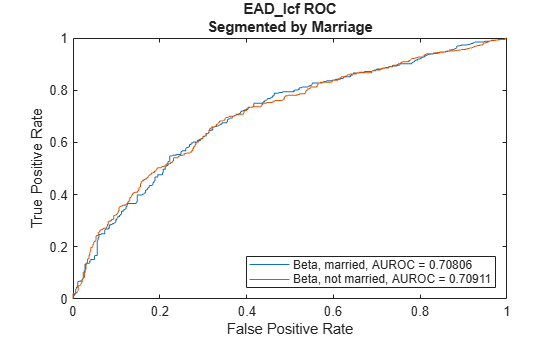## Input Arguments

collapse all

Exposure at default model, specified as a previously created `Regression`, `Tobit`, or `Beta` object using `fitEADModel`.

Data Types: `object`

Data, specified as a `NumRows`-by-`NumCols` table with predictor and response values. The variable names and data types must be consistent with the underlying model.

Data Types: `table`

### Name-Value Arguments

Specify optional pairs of arguments as `Name1=Value1,...,NameN=ValueN`, where `Name` is the argument name and `Value` is the corresponding value. Name-value arguments must appear after other arguments, but the order of the pairs does not matter.

Example: ```[DiscMeasure,DiscData] = modelDiscrimination(eadModel,data(TestInd,:),DataID='Testing',DiscretizeBy='median')```

Data set identifier, specified as `DataID` and a character vector or string. The `DataID` is included in the output for reporting purposes.

Data Types: `char` | `string`

Discretization method for EAD `data` at the defined `ModelLevel`, specified as `DiscretizeBy` and a character vector or string.

• `'mean'` — Discretized response is `1` if observed EAD is greater than or equal to the mean EAD, `0` otherwise.

• `'median'` — Discretized response is `1` if observed EAD is greater than or equal to the median EAD, `0` otherwise.

Data Types: `char` | `string`

Name of a column in the `data` input, not necessarily a model variable, to be used to segment the data set, specified as `SegmentBy` and a character vector or string. One AUROC is reported for each segment, and the corresponding ROC data for each segment is returned in the optional output.

Data Types: `char` | `string`

Indicates if the output includes columns showing segment value and segment count, specified as the comma-separated pair consisting of `'ShowDetails'` and a scalar logical.

Data Types: `logical`

Model level, specified as `ModelLevel` and a character vector or string.

Note

`Regression` models support all three model levels, but a `Tobit` or `Beta` model supports only a `ModelLevel` for `"ead"` and `"conversionMeasure"`.

Data Types: `char` | `string`

EAD values predicted for `data` by the reference model, specified as `ReferenceEAD` and a `NumRows`-by-`1` numeric vector. The `modelDiscrimination` output information is reported for both the `eadModel` object and the reference model.

Data Types: `double`

Identifier for the reference model, specified as `ReferenceID` and a character vector or string. `'ReferenceID'` is used in the `modelDiscrimination` output for reporting purposes.

Data Types: `char` | `string`

## Output Arguments

collapse all

AUROC information for each model and each segment, returned as a table. `DiscMeasure` has a single column named `'AUROC'` and the number of rows depends on the number of segments and whether you use a `ReferenceID` for a reference model . The row names of `DiscMeasure` report the model IDs, segment, and data ID. If the optional `ShowDetails` name-value argument is `true`, the `DiscMeasure` output displays `Segment` and `SegmentCount` columns.

Note

If you do not specify `SegmentBy` and use `ShowDetails` to request the segment details, the two columns are added and show the `Segment` column as `"all_data"` and the sample size (minus missing values) for the `SegmentCount` column.

ROC data for each model and each segment, returned as a table. There are three columns for the ROC data, with column names `'X'`, `'Y'`, and `'T'`, where the first two are the X and Y coordinates of the ROC curve, and T contains the corresponding thresholds. For more information, see Model Discrimination or `perfcurve`.

If you use `SegmentBy`, the function stacks the ROC data for all segments and `DiscData` has a column with the segmentation values to indicate where each segment starts and ends.

If reference model data is given, the `DiscData` outputs for the main and reference models are stacked, with an extra column `'ModelID'` indicating where each model starts and ends.

collapse all

### Model Discrimination

Model discrimination measures the risk ranking.

The `modelDiscrimination` function computes the area under the receiver operator characteristic (AUROC) curve, sometimes called simply the area under the curve (AUC). This metric is between 0 and 1 and higher values indicate better discrimination.

To compute the AUROC, you need a numeric prediction and a binary response. For EAD models, the predicted EAD is used directly as the prediction. However, the observed EAD must be discretized into a binary variable. By default, observed EAD values greater than or equal to the mean observed EAD are assigned a value of 1, and values below the mean are assigned a value of 0. This discretized response is interpreted as "high EAD" vs. "low EAD." Therefore, the `modelDiscrimination` function measures how well the predicted EAD separates the "high EAD" vs. the "low EAD" observations. You can change the level to compute the model discrimination with the `ModelLevel` name-value pair argument and the discretization criterion with the `DiscretizeBy` name-value pair argument.

To plot the receiver operator characteristic (ROC) curve, use the `modelDiscriminationPlot` function. However, if you need the ROC curve data, use the optional `DiscData` output argument from the `modelDiscrimination` function.

The ROC curve is a parametric curve that plots the proportion of

• High EAD cases with predicted EAD greater than or equal to a parameter t, or true positive rate (TPR)

• Low EAD cases with predicted EAD greater than or equal to the same parameter t, or false positive rate (FPR)

The parameter t sweeps through all the observed predicted EAD values for the given data. The `DiscData` optional output contains the TPR in the `'X'` column, the FPR in the `'Y'` column, and the corresponding parameters t in the `'T'` column. For more information about ROC curves, see ROC Curve and Performance Metrics.

 Baesens, Bart, Daniel Roesch, and Harald Scheule. Credit Risk Analytics: Measurement Techniques, Applications, and Examples in SAS. Wiley, 2016.

 Bellini, Tiziano. IFRS 9 and CECL Credit Risk Modelling and Validation: A Practical Guide with Examples Worked in R and SAS. San Diego, CA: Elsevier, 2019.

 Brown, Iain. Developing Credit Risk Models Using SAS Enterprise Miner and SAS/STAT: Theory and Applications. SAS Institute, 2014.

 Roesch, Daniel and Harald Scheule. Deep Credit Risk. Independently published, 2020.